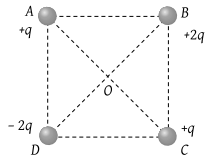The law, governing the force between electric charges is known as

(1) Ampere's law

(2) Ohm's law

(4) Coulomb's law

Subtopic:  Coulomb's Law |
To view explanation, please enrol in the course below.
NEET 2021 - Achiever Batch - Aryan Raj Singh

Difficulty Level:

Fg and Fe represents gravitational and electrostatic force respectively between electrons situated at a distance 10 cm. The ratio of Fg/ Fe is of the order of

(1) 1042

(2) 10

(3) 1

(4) 10–43

Subtopic:  Coulomb's Law |
To view explanation, please enrol in the course below.
NEET 2021 - Achiever Batch - Aryan Raj Singh

Difficulty Level:

Four charges are arranged at the corners of a square ABCD, as shown in the adjoining figure. The force on the charge kept at the centre O is(1) Zero

(2) Along the diagonal AC

(3) Along the diagonal BD

(4) Perpendicular to side AB

Subtopic:  Coulomb's Law |
To view explanation, please enrol in the course below.
NEET 2021 - Achiever Batch - Aryan Raj Singh

Difficulty Level:

In the absence of other conductors, the surface charge density

(1) Is proportional to the charge on the conductor and its surface area

(2) Inversely proportional to the charge and directly proportional to the surface area

(3) Directly proportional to the charge and inversely proportional to the surface area

(4) Inversely proportional to the charge and the surface area

Subtopic:  Electric Field |
To view explanation, please enrol in the course below.
NEET 2021 - Achiever Batch - Aryan Raj Singh

Difficulty Level:

Out of gravitational, electromagnetic, Vander Waals, electrostatic and nuclear forces; which two are able to provide an attractive force between two neutrons

(1) Electrostatic and gravitational

(2) Electrostatic and nuclear

(3) Gravitational and nuclear

(4) Some other forces like Vander Waals

Subtopic:  Coulomb's Law |
To view explanation, please enrol in the course below.
NEET 2021 - Achiever Batch - Aryan Raj Singh

Difficulty Level:

Three charges 4q, Q, and q are in a straight line in the position of 0, l/2, and l respectively. The resultant force on q will be zero if Q =

(1) – q

(2) –2q

(3) $-\frac{q}{2}$

(4) 4q

Subtopic:  Coulomb's Law |
To view explanation, please enrol in the course below.
NEET 2021 - Achiever Batch - Aryan Raj Singh

Difficulty Level:

Two small spheres each having the charge +Q are suspended by insulating threads of length L from a hook. This arrangement is taken in space where there is no gravitational effect, then the angle between the two suspensions and the tension in each will be

(1) ${180}^{o},\text{\hspace{0.17em}}\frac{1}{4\pi {\epsilon }_{0}}\frac{{Q}^{2}}{{\left(2L\right)}^{2}}$

(2) ${90}^{o},\text{\hspace{0.17em}}\frac{1}{4\pi {\epsilon }_{0}}\frac{{Q}^{2}}{{L}^{2}}$

(3) ${180}^{o},\text{\hspace{0.17em}}\frac{1}{4\pi {\epsilon }_{0}}\frac{{Q}^{2}}{2{L}^{2}}$

(4) ${180}^{o},\text{\hspace{0.17em}}\frac{1}{4\pi {\epsilon }_{0}}\frac{{Q}^{2}}{{L}^{2}}$

Subtopic:  Coulomb's Law |
To view explanation, please enrol in the course below.
NEET 2021 - Achiever Batch - Aryan Raj Singh

Difficulty Level:

Two charges each of 1 coulomb are at a distance 1 km apart, the force between them is

(1) 9 × 103 Newton

(2) 9 × 10–3 Newton

(3) 1.1 × 10–4 Newton

(4) 104 Newton

Subtopic:  Coulomb's Law |
To view explanation, please enrol in the course below.
NEET 2021 - Achiever Batch - Aryan Raj Singh

Difficulty Level:

+2C and +6C two charges are repelling each other with a force of 12 N. If each charge is given –2C of charge, then the value of the force will be

(1) 4 N (Attractive)

(2) 4 N (Repulsive)

(3) 8 N (Repulsive)

(4) Zero

Subtopic:  Coulomb's Law |
To view explanation, please enrol in the course below.
NEET 2021 - Achiever Batch - Aryan Raj Singh

Difficulty Level:

The dielectric constant of pure water is 81. Its permittivity will be

(1) 7.17 × 10–10 MKS units

(2) 8.86 × 10–12 MKS units

(3) 1.02 × 1013 MKS units

(4) Cannot be calculated

Subtopic:  Coulomb's Law |
To view explanation, please enrol in the course below.
NEET 2021 - Achiever Batch - Aryan Raj Singh

Difficulty Level: